# Bihar Board 12th Maths Model Question Paper 3 in English Medium

Bihar Board 12th Maths Model Papers

## Bihar Board 12th Maths Model Question Paper 3 in English Medium

Time : 3 Hours 15 Min
Full Marks: 100

Instructions for the candidates :

1. Candidates are required to give their answers in their own words as far as practicable.
2. Figure in the right-hand margin indicates full marks.
3. While answering the questions, the candidate should adhere to the word limit as far as practicable.
4. 15 Minutes of extra time has been allotted for the candidate to read the questions carefully.
5. This question paper is divided into two sections. Section-A and Section-B
6. In Section A, there are 1-50 objective type questions which are compulsory, each carrying 1 mark. Darken the circle with blue/black ball pen against the correct option on the OMR Sheet provided to you. Do not use Whitener/Liquid/ Blade/Nail on OMR Sheet otherwise result will be invalid.
7. In section-B, there are 25 short answer type questions (each carrying 2 marks), out of which only 15 (fifteen) questions are to be answered.
A part from this there is 08 Long Answer Type questions (each carrying 5 marks), out of which 4 questions are to be answered.
8. Use of any electronic device is prohibited.

Objective Type Questions

There are 1 to 50 objective type questions with 4 options, choose the correct option which is to be answered on OMR Sheet. (50 × 1 = 50)

Question 1.
Let R be the relation in the set N given by R = {(a, b): a = b – 2, b > 6}. Which of the following is correct answer.
(a) (2,4) ∈ R
(b) (3,8) ∈ R
(c) (6,8) ∈ R
(d) (8,7) ∈ R
(c) (6,8) ∈ RQuestion 2.
Let f: R → R be defined as f(x) = x4. Choose the correct answer ?
(a) f is one-one and onto
(b) f is many one onto
(c) f is one-one but not onto
(d) f is neither one-one nor opnto
(d) f is neither one-one nor opnto

Question 3.
Let a binary operation on N defined as L.C.M. of a * b = a and b. Then (20 * 16) – (16 * 20) is equal to
(a) 4
(b) 60
(c) 0
(d) 1
(c) 0

Question 4.
The value of $$\sin \left(\frac{\pi}{3}-\sin ^{-1}\left(-\frac{1}{2}\right)\right)$$ is
(a) $$\frac{1}{2}$$
(b) $$\frac{1}{3}$$
(c) $$\frac{1}{4}$$
(d) 1
(d) 1Question 5.
The principle value of $$\sin ^{-1}\left(\frac{\sqrt{3}}{2}\right)$$ is
(a) $$\frac{2 \pi}{3}$$
(b) $$\frac{\pi}{6}$$
(c) $$\frac{\pi}{4}$$
(d) $$\frac{\pi}{3}$$
(d) $$\frac{\pi}{3}$$

Question 6.
tan-1 =
(a) cos-1x
(b) $$\frac{1}{\cot ^{-1} x}$$
(c) $$\cot ^{-1} \frac{1}{x} 0$$
(d) -cot-1x
(b) $$\frac{1}{\cot ^{-1} x}$$

Question 7.
The number of all possible matrices of order 3 x 3 with each entry 0 or 1.
(a) 27
(b) 18
(c) 81
(d) 512
(d) 512

Question 8.
If A = $$\left[\begin{array}{cc} \alpha & \beta \\ \gamma & -\alpha \end{array}\right]$$ such that A2 = I,
(a) 1 + α2 + βγ = 0
(b) 1 – α2+ βγ = 0
(c) 1 – α2 – βγ = 0
(d) 1 + α2 – βγ = 0
(c) 1 – α2 – βγ = 0Question 9.
The value of determinant $$\left|\begin{array}{lll} \frac{1}{a} & 1 & b c \\ \frac{1}{b} & 1 & c a \\ \frac{1}{c} & 1 & a b \end{array}\right|$$ is
(a) 0
(b) abc
(c) 1/abc
(d) None of these
(a) 0

Question 10.
The value of determinant is $$\left|\begin{array}{lll} 2 & 7 & 65 \\ 3 & 8 & 75 \\ 5 & 9 & 86 \end{array}\right|$$ is
(a) 0
(b) 1
(c) 2
(d) 3
(a) 0

Question 11.
$$\left|\begin{array}{lll} 1 & x & x^{2} \\ 1 & y & y^{2} \\ 1 & z & z^{2} \end{array}\right|=$$
(a) (x – y) (y + z) (z + x)
(b) (x+y) (y – z) (z – x)
(c) (x – y)(y – z) (z + x)
(d) (x – y)(y – z) (z – x)
(d) (x – y)(y – z) (z – x)Question 12.
If , $$\left[\begin{array}{cc} 2 x-y & 5 \\ 3 & y \end{array}\right]=\left[\begin{array}{cc} 6 & 5 \\ 3 & -2 \end{array}\right]$$ then x =
(a) 3
(b) 4
(c) 5
(d) 8
(b) 4

Question 13.
If $$f(x)=\left\{\begin{array}{l} x, \text { if } x \geq 0 \\ 0, \text { if } x<0 \end{array}\right.$$ then the function is
(a) Continuous
(b) Discontinuous
(c) well defined
(d) None of these
(b) Discontinuous

Question 14.
In how many points the function f(x) = $$\frac{1}{\log |x|}$$ is discontinuous
(a) 1
(b) 2
(c) 3
(d) 4
(c) 3Question 15.
The differential of sin (x2 + 5) is
(a) 2 sin (x + 5)
(b) 2 cos (x2 + 5)
(c) 2x cos (x2 + 5)
(d) None of these
(c) 2x cos (x2 + 5)

Question 16.
If y = log x then the value of $$\frac{d y}{d x}$$ is
(a) x log x
(b) $$\frac{1}{x \log x}$$
(c) $$\frac{1}{\log x}$$
(d) $$\frac{1}{ x }$$
(d) $$\frac{1}{ x }$$

Question 17.
If y = (3x2 – 9x + 5)9 then $$\frac{d y}{d x}$$ is equal to :
(a) 27 (3x2 – 9x + 5)8 (2x – 3)
(b) 27 (3x2 – 9x + 5)
(c) 27 (2x – 3)
(d) None of these
(a) 27 (3x2 – 9x + 5)8 (2x – 3)

Question 18.
The rate of change the area of circle w.r.t.r, when r = 5 cm.
(a) 10πcm2/cm
(b) 12πcm2/cm
(c) 8π cm2/cm
(d) 11πcm2/cm
(a) 10πcm2/cm

Question 19.
The function f(x) = sin x is strictly increasing in the interval
(a) (0,1)
(b) $$\left(\frac{\pi}{2}, \pi\right)$$
(c) $$\left(0, \frac{\pi}{2}\right)$$
(d) None of these
(c) $$\left(0, \frac{\pi}{2}\right)$$Question 20.
The function f(x) = e2x is
(a) strictly increasing
(b) strictly decreasing
(c) neither incrasing nor decreasing
(d) None of these
(a) strictly increasing

Question 21.
The slope of tangent to the curve y = x2 + 3r + 4 at (1,1) is
(a) 5
(b) $$-\frac{1}{5}$$
(c) 8
(d) None of these
(b) $$-\frac{1}{5}$$

Question 22.
The line y = mx is a tangent to the curve y2 = 4r if the value of m is
(a) 1
(b) 2
(c) 3
(d) $$\frac{1}{2}$$
(a) 1

Question 23.
If f(x) = 3x2 + 15x + 5, then approximate value of f(3.02) is
(a) 47.66
(b) 57.66
(c) 67.66
(d) 77.66
(d) 77.66Question 24.
The antiderivative of (ax + b)2 is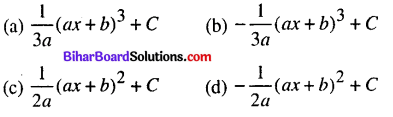$$\frac{1}{3 a}(a x+b)^{3}+C$$

Question 25.
$$\int \frac{\sin ^{2} x-\cos ^{2} x}{\sin ^{2} x \cos ^{2} x} d x$$ is equal to
(a) tan x + cot x + C
(b) tan x + cosec x + C
(c) – tan x + cot x + C
(d) tan x + sec x + C
(a) tan x + cot x + C

Question 26.
∫ log xdx is equal to
(a) xlogx + x + C
(b) xlogx – x + C
(c) log x + x + C
(d) logx – x + C
(b) xlogx – x + C

Question 27.
If f(a + b – x) = f(x) then $$\int_{a}^{b} x f(x) d x$$ is equal to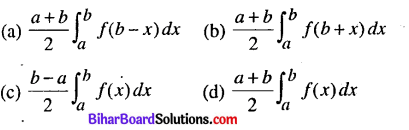$$\frac{a+b}{2} \int_{a}^{b} f(x) d x$$

Question 28.
The value of $$\int_{0}^{1} \tan ^{-1}\left(\frac{x}{1+x^{2}}\right) d x$$ is
(a) 1
(b) $$\frac{\pi^{2}}{32}$$
(c) -1
(d) $$\frac{\pi}{4}$$
(b) $$\frac{\pi^{2}}{32}$$Question 29.
$$\int_{0}^{1} \tan ^{-1}\left(1-x+x^{2}\right) d x$$ is equal to
(a) log 2
(b) $$\log \frac{1}{2}$$
(c) πlog2
(d) $$\frac{\pi}{2} \log \frac{1}{2}$$
(c) πlog2

Question 30.
The area enclosed by circle x2 + y2 = a2 is
(a) 4
(b) πa2
(c) 2
(d) 1
(b) πa2

Question 31.
The order and degree of $$\left(\frac{\mathrm{ds}}{\mathrm{dt}}\right)^{4}+3 \mathrm{s} \frac{\mathrm{d}^{2} \mathrm{s}}{\mathrm{dt}^{2}}=0$$ is
(a) 1, 2
(b) 2, 1
(c) 2, 4
(d) 4, 2
(b) 2, 1

Question 32.
Which of the following is linear differential equation ?
(a) (4x + 6y + 5) dy – (3y + 2x + 4)dx = Q
(b) xydx – (x3 + y3) dy = 0
(c) (x3 + 2y2) dx + 2xydy = 0
(d) y2dx + (x2 – xy – y2) dy = 0
(d) y2dx + (x2 – xy – y2) dy = 0Question 33.
The integrating factor at the diff eqn (1 – y2) $$\frac{d x}{d y}$$ + yx = ay (-1 < y < -1) is(d) $$\frac{1}{\sqrt{1-y^{2}}}$$

Question 34.
The general solution of diff eq $$\frac{y d x-x d y}{y}=0$$ is
(a) xy = C
(b) x = cy2
(c) y = cx
(d) y = cx2
Answre:
(c) y = cx

Question 35.
The generval solution of diff. eqn exdy + (yex + 2x)dx = 0 is
(a) xex + x2 = C
(b) xey + y2 = C
(c) yex + x2 = C
(d) yex + x2 = C
(c) yex + x2 = CQuestion 36.
If the adjacent side of parallelogram are $$\vec{a}$$ and $$\vec{b}$$ then its area is(a) $$|\vec{a} \times \vec{b}|$$

Question 37.
If a + b + c = 0 then
(a) $$\vec{a} \cdot \vec{b}=0$$
(b) $$\vec{a}=\vec{b}$$
(c) $$\vec{a} \times \vec{b}=\vec{b} \times \vec{c}=\vec{c} \times \vec{a}$$
(d) None of these
(c) $$\vec{a} \times \vec{b}=\vec{b} \times \vec{c}=\vec{c} \times \vec{a}$$

Question 38.
If $$\vec{a}$$ and $$\vec{b}$$ are two vectors then $$(\vec{a}+\vec{b}) \cdot(\vec{a}-\vec{b})$$ is equal to(d) $$2(a \times b)$$

Question 39.
The angle between the vectors 2î – 3ĵ + 2k̂ and î + 4ĵ + 5k̂ is
(a) 30°
(b) 90°
(c) 45°
(d) 60°
(b) 90°

Question 40.
If θ be the angle between the vectors $$\vec{a}$$ and $$\vec{b}$$ then sin θ is(a) $$\frac{\vec{a} \cdot \vec{b}}{|\vec{a} \| \vec{b}|}$$

Question 41.
The mean and variance of a binomial distribution are 6 and 1 respectively. Then the value of parameter n is
(a) 18
(b) 12
(c) 10
(d) 9
(a) 18Question 42.
The plane x = 0,y = 0 is
(a) Parattel
(b) Perpendicular
(c) Intersect z-axis
(d) None of these
(c) Intersect z-axis

Question 43.
Linear programming problem is with
(a) One objective function
(b) Two objective function
(c) No objective function
(d) None of these
(a) One objective function

Question 44.
If P(A) = 0.8, P(B) = 0.5 and P(B/A) = 0.4 then P(A/B) =
(a) 0.32
(b) 0.64
(c) 0.16
(d) 0.25
(b) 0.64

Question 45.
The mean and variance of a random X are 4 and 2 respectively P(X = 1) is
(a) 1/32
(b) 1/16
(c) 1/8
(d) 1/4
(b) 1/16Question 46.
The pair of dice are rolled. The probability of obtaining an even prime number on each die is
(a) 1/36
(b) 1/12
(c) 1/6
(d) 0
(a) 1/36

Question 47.
If P(A∪B) = 0.8 and P(A∩B) = 0.3 then P(Ā) + P(B̄) =
(a) 0.3
(b) 0.5
(c) 0.7
(d) 0.9
(a) 0.3

Question 48.
Let the binary operation * on R defined by x *y = 1 + 12x + xy ∀ x,y ∈ R then 2 * 3 is
(a) 31
(b) 41
(c) 43
(d) 51
(a) 31

Question 49.
Which of the following is the equation of ary – plane
(a) x = 0
(b) y = 0
(c) x = k
(d) z = 0
(a) x = 0Question 50.
In a hostel 60 % of the students read Hindi news paper, 40% read. English news paper and 20% read both Hindi and English news paper. Then what is probability that reads neither Hindi nor English news paper
(a) 0.2
(b) 0.3
(c) 0.4
(d) 0.5
(a) 0.2

Non – Objective Type Questions

Q. No. 1 to 25 are of Short Answer type carry of 2 marks each. Answer any 15 questions. (15 × 2 = 30)

Question 1.
Let N be the set of natural numbers and relation R define one N. as R = {(x,y); x,y ∈ N and x divide y} Verify that the whether R is reflexive, symmetric and transitive.
Let.x, y, z, ∈ N
(i) xR.x ⇒ x divide x i.e. xRx. ∀ x ∈ N. So R is reflexive.
(ii) x divide y ⇒ xRy ⇒ (x, y) ∈ R but (y, x) ∉ R. Since .x divide y therefore it is not possible y divide x due to x, y ∈ N.SoR is not symmetric
(iii) x divide y, y divide z ⇒ x divide z i.e. .xRy, yRz ⇒ yRz So R is transitive.

Question 2.
Show that the function f: N → N defined as f(x) = 2x is one-one but not onto.
we have f(x) = 2x ⇒ f(x1) = f(x2) ⇒ 2x1 = 2x2
⇒ x1 = x2 so, f is one-one
Further,f is not onto, as for 1 ∈ N, there does not exist any x in N such that f(x) = 2x = 1 ∈ N.
Thus f is one-one but not onto

Question 3.
Prove that the binary opeation * on the set N such that a * b = $$\frac{a+b}{2}$$ ∀ a,b,∈ N is commutative but not associative.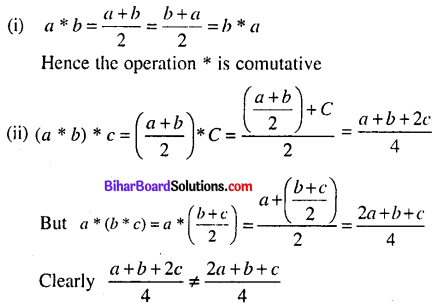Thus the binary operation * is commutative but not associativeQuestion 4.
Find the value of cot [tan-1 a + cot-1 a]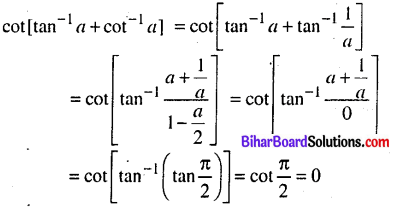Question 5.
Prove that $$\sin ^{-1} \frac{2 \sqrt{2}}{3}+\sin ^{-1} \frac{1}{3}=\frac{\pi}{2}$$Question 6.
Find the value of x if $$\left[\begin{array}{ll} x & 4 \\ 2 & 2 x \end{array}\right]=0$$
$$\left[\begin{array}{ll} x & 4 \\ 2 & 2 x \end{array}\right]=0$$
⇒ 2x2 = 8 ⇒ x2 = 4 ⇒ x = ±2Question 7.
If A = $$\left[\begin{array}{lll} 3 & \sqrt{3} & 2 \\ 4 & 2 & 0 \end{array}\right]$$ then prove that (A’)’ = A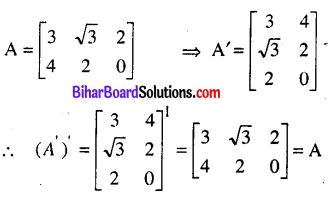Question 8.
Find the value at x if $$\left|\begin{array}{ll} 3 & x \\ x & 1 \end{array}\right|=\left|\begin{array}{ll} 3 & 2 \\ 4 & 1 \end{array}\right|$$
$$\left|\begin{array}{ll} 3 & x \\ x & 1 \end{array}\right|=\left|\begin{array}{ll} 3 & 2 \\ 4 & 1 \end{array}\right|$$
⇒ 3 – 2x2 = 3- 8 ⇒ x2 = 8
∴ x = ±2√2

Question 9.
Find the value of x and y if $$\left|\begin{array}{ll} 2 & 3 \\ y & x \end{array}\right|=4$$ and $$\left|\begin{array}{ll} x & y \\ 2 & 1 \end{array}\right|=\frac{7}{2}$$⇒ y = -3
Multiplying (2) by 2 and subtract (1), we get Putting the value of y in (2), we getQuestion 10.
If a matrix has 24 elements. What are the possible orders it can have ? what, if has 13 element.
The possible order of matrices for 24 elements are :
1 × 24, 24 × 1,2 × 12, 12 × 2, 3 × 8,8 × 3, 4 x 6 and 6 × 4
Again, possible order of matrices for 13 elements are 1 × 13 and 13 × 1.

Question 11.
Verify the Rolle’s theorem of the function f(x) = x3 (x – 1)2 for the interval [0,1)
Given, f(x) = 3(x – 1)2
⇒ f'(x) = 3x2 (x-1)2 + x3 2(x – 1) = x2 (x – 1) (3 (x – 1) + 2x) or, f'(x) = x2 (x – 1) (5x – 3)
clearly f(x) is differentiable and continuous for each x.
(ii) f(x) is differentiable in (0, 1)
(iii) f(0) = 0 and f(1) = 0, ∴ f(0) =f(1)
So, all the condition of the Rolles theorem are satisfies for the function f(x) in (0, 1). Then f'(c) = 0
⇒ c2(c – 1)(5c – 3) = 0 c = 0,1,3/5
But 0< c < 1 ∴ c = 3/5

Question 12.
If x = at2, y = 2at. then find that dy/dx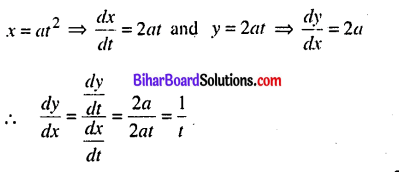Question 13.
Find the anti derivative F off defined by f(x) = 4x3 – 6, where F(0) = 3.
one anti-derivative of f(x) is x4 – 6x since
$$\frac{d}{d x}$$ (x4-6x) = 4x3 – 6
Therefore, the anti derivative F is given by
F(x) = x4 – 6x + C, where C is constant
Given that F(0) = 3, which gives 3 = 0 – 6 × 0 + C
⇒ C = 3
Thus, the required anti-derivative is the unique function F defined by F(x) = x4 – 6x + 3Question 14.
Evaluate: $$\int_{0}^{1} x(1-x)^{23} d x$$
Let z = 1 – x, then dz = -dx and x = 1 – z
when x = 0, z = 1 and when x = 1, z = 0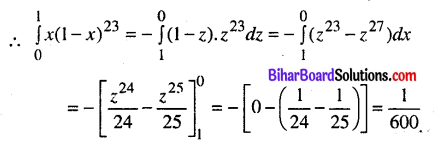Question 15.
Evaluate: $$\int_{a}^{b} \frac{\log x}{x} d x$$Question 16.
Find the differential equation of the curve y = Aex + Be-x; where A and B are constant.
y = Aex + Be-x
Differentiating both side w.r.t. x, we get
$$\frac{d y}{d x}$$ = Aex + Be-x
Again, differentiating w.r.t we getor, $$\frac{d^{2} y}{d x^{2}}-y=0$$ which is required, differential equation.

Question 17.
Find the particular solution of the differential equation $$\frac{d y}{d x}$$ = ytanx when y = 1 if x = 0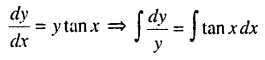⇒ logy = -logcos x+ logC
⇒ log y + logcosx = log C
⇒ log (ycosx) = logC ⇒ ycosx = C …….(1)
Now, putting y= I and x = 0 in (1), we get
cos 0 = C ⇒ C = 1 ∴ y cos x = 1
y = $$\frac{1}{\cos x}$$ ⇒ y = sec xQuestion 18.
Find the unit vector in the direction of the vector $$\overrightarrow{\mathrm{a}}=2 \hat{\mathrm{i}}+3 \hat{\mathrm{j}}+\hat{\mathrm{k}}$$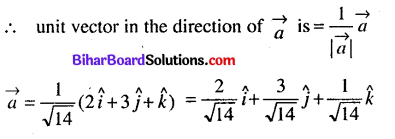Question 19.
If $$\overrightarrow{\mathrm{a}}=2 \hat{\mathrm{i}}+\hat{\mathrm{j}}+3 \hat{\mathrm{k}}$$ and $$\overrightarrow{\mathbf{b}}=3 \hat{\mathbf{i}}+5 \hat{\mathbf{j}}-2 \hat{\mathbf{k}}$$ then find the $$|\overrightarrow{\mathrm{a}} \times \overrightarrow{\mathrm{b}}|$$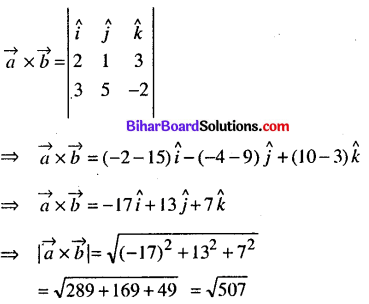Question 20.
Find the equation of the planes parallel to the plane dr -3y + 2z + 9 = 0 and at distance 5 from the origin.
Let the plane 6x – 3y + 2z + k = 0 is the parallel, to the plane 6x – 3y + 2z + 9 = 0. Now, the distance of plane 6x – 3y + 2z + 9 = 0 from the origin isThus the plane is 6x – 3y + 2k ± 35 = 0

Question 21.
A Player has 7 cards in hand of which 5 arc red and of these five 2 are kings. A card is drawn at random. Find the probability that it is a king, it is known that it is red.
Let A = The event of red card and B = The event of king.
Then n(A) = 5 and the events are red and king is = A ∩ B
∴ n(A) = 5 and the events of red and king – A∩B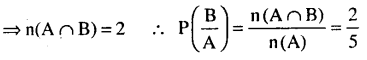Question 22.
Find the probability of the occurrence of a number greater than 1 if it is known that only odd numbers can occur in a die.
Let A = The event of occurrence odd number and B = the event of occurrence the odd number greater Then
Then A = {1, 3,5} ⇒ n(A) = 3 and B = {3,5)
then A ∩ B = {3,5} ⇒ n(A∩B) = 2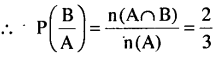Question 23.
Verify the continuity of the function f(x) = 2x2 – 1 at x = 3.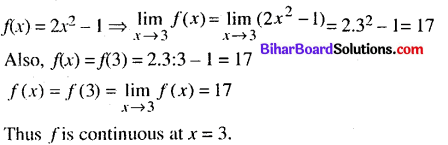Question 24.
The radius of a circle is increasing at the rate of 0.7 cm/s. What is the rate of increase of its circumference when r = 4.9 cm ?
Let c and r be the circumference and radius of circle. Then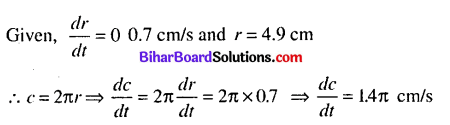Question 25.
Find the slope of the tangent to the curvey = x3 – x + 1 at the point whose x-co-ordinate is 2.
Given the curve y = x3 – x + 1Questions No. 26 to 33 are Long Answer Type Questions. Each question carry 5 marks. Answer any 4 question. (4 × 5 = 20)

Question 26.
Prove that of all the rectangles inseribed in a given circle the square has the maximum area.
Let ABCD be a rectangle inscribed in a given circle of centre O and radius r.
Let AB = 2x and BC = 2y,
∴∆ AOAM be a right angled triangle. Then
OA2 = AM2 + OM2
or, a2 = x2 + y2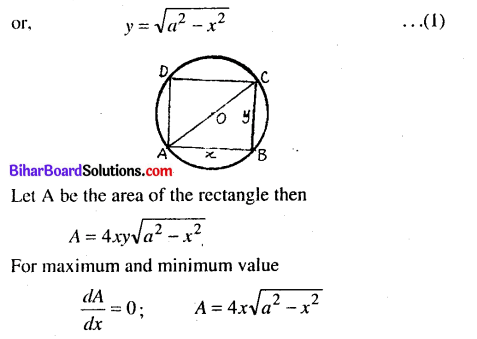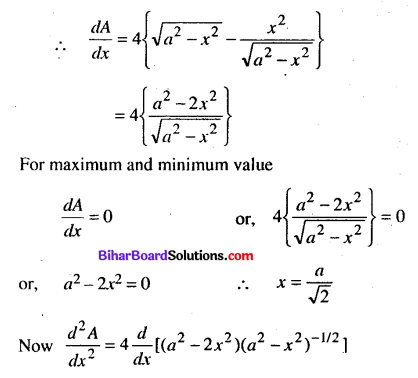Hence area is maximum when x = y
or, 2x = 2y Thus the rectangle is square.Question 27.
Verify Lagrange’s mean value theorem when the function f(x) = (x – 1) (x – 2) (x – 3) in the interval [0,4].
The given function, f(x) = (x – 1)(x – 2)(x – 3) in interval [0,4]
⇒ f(x) = (x2 -x -2x + 2) (x – 3)
⇒ f(x) = (x2 – 3x + 2) (x – 3) = x3 – 3x2 + 2x – 3x2 + 9x – 6
⇒ f(x) = x3 -6x2 + 11x – 6
Since f(x) is a polynomial function and polynomial function is continuous, i.e. f(x) is continuous in [0, 4]
Also, f'(x) = 3x2 – 2x +11, exists in interval ]0, 4[.
So, f(x) is differentiable in ]0,4[. Thus Lagrange’s theorem are satisfied
∴ c ∈] 0,4 [ exist.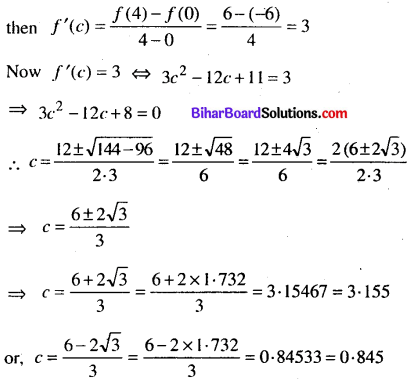Clearly, the both value of c lie between ] 0, 4 [. Thus theorem is verified.

Question 28.
Find area enclosed by the ellipse $$\frac{x^{2}}{9}+\frac{y^{2}}{4}=1$$
We have, The equation of ellipsePutting x = 3sinθ ⇒ dx = 3cosθ. Also,
when x = 0, then θ = 0 and when A = 3 then θ = π/2
∴ Required area of the ellipse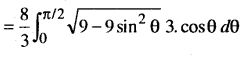Question 29.
Evaluate: $$\int_{0}^{\pi / 2} \log \sin x d x$$Question 30.
Prove that
$$\overrightarrow{[a} \times \vec{b}, \vec{b} \times \vec{c}, \vec{c} \times \vec{a}]=[\vec{a}, \vec{b}, \vec{c}]^{2}$$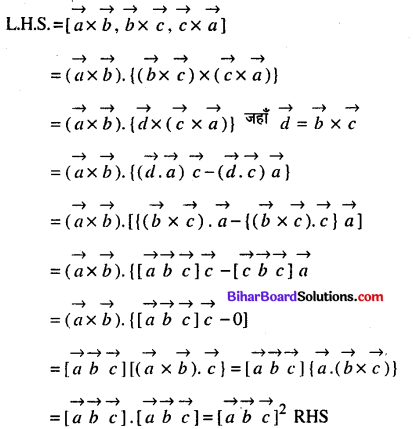Question 31.
Find the co-ordinates of the foot of the perpendicular drawn from the origin to the plane 2r – 3y + 4z – 6 = 0.
Let the co-ordinates of the foot of the perpendicular P from the origin to the plane is P (x1, y1, z1).
Then the direction ratios of the line OP are x1y1, z1.
Now the direction ratio of the plane is 2, – 3, 4. So direction cosines are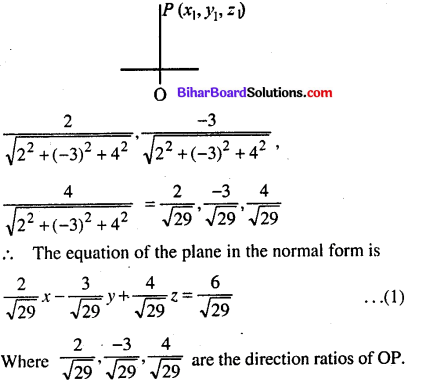Now, since the direction cosines and direction ratios of the line are proportional. Then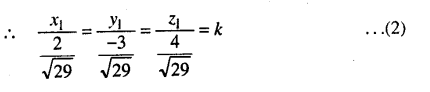Thus the co-ordinates of foot of perpendicular isQuestion 32.
Solve the LPP: Minimize Z = – 3x + 4x
under the constraints x + 2y ≤ 8, 3x + 2y ≤ 12, x ≥ 0, y ≥ 0.
Minimize Z = -3x + 4. under the costraints
A + 2y ≤ 8 ⇒ x + 2y = 8 …..(1)
3x + 2y ≤ 12 ⇒ 3x + 2y = 12 …(2)
x ≥ 0, y ≥ 0 ⇒ x = 0, y = 0 …(3)First of all draw the graph of the equations (1) to (3) corresponds to the inequations. It is clear from figure, that (1) and (2) are intersect each other at the point (2,3). So, we get a feasible region OABC, which is bounded. The co-ordinates of the corner points of the feasible region are 0(0, 0) A(4,0), B(2, 3) and C (0, 4). Lastly, applying corner point method to find the value of the objective function Z are as follows :It is clear from the above table that the minimum value of Z at the point (4,0) is – 12. Thus the minimum value of Z is -12Question 33.
A furniture dealer deals in only two items tables and chairs. He has Rs. 5000/- to invest and a space to store at most 60 pices. A table costs him Rs. 250 and a chair Rs. 50. He can sell a table at a profit to Rs. 50 and a chair at a profit of Rs. 15. Assuming that he can sell all the items that he buys how should he invest his money in order that he may maximize his profit.
Let x and y represents tables and chairs respectively. Now, by question costs of x tables = 250 x and the chair = 50y ∴ Total costs (250x + 50y)
Since the dealer has invest Rs. 5000
∴ 250x + 50v ≤ 5000 =>5y + y ≤ 100
Again, the dealer has a space to store at most 60, so
x + y ≤ 60
Also, non-negative constraints : x ≥ 0, y ≥ 0.
Further, profit from x table = 50.v and y chair = 15y
Objective Function Z = 50x + 15y
So. the mathematical form of the problem are as follows
Maximize Z = 50x + 15y
Subject to constraints 5x + y ≤ 100 ⇒ 5x + y = 100 .. .(1)
v + y ≤ 60 ⇒ x + y = 60 .. .(2)
x ≥ 0, y ≥ 0 ⇒  x = 0, y = 0 …(3)
First of all draw the graph of equations (1) to (3) corresponds to inequations. On joining the points (20, 0),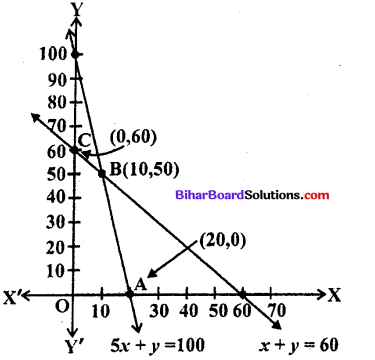(0,100) and (60,0), (0,60) we get two straight line, which intersects at the point (10, 50). The point of intersection (10, 50) can be obtained from solving equations (1) and (2). It is clear from figure OABC be the feasible region, which is bounded. Lastly, by the using comer points of feasible region 0(0, 0), A(20,0), B(10,50) and C(0, 60) to find the maximum values of Z :It is clear from the table that the maximum value of Z is Rs. 1250. Thus the maximum profit to the dealer for buying 10 tables and 50 chairs.